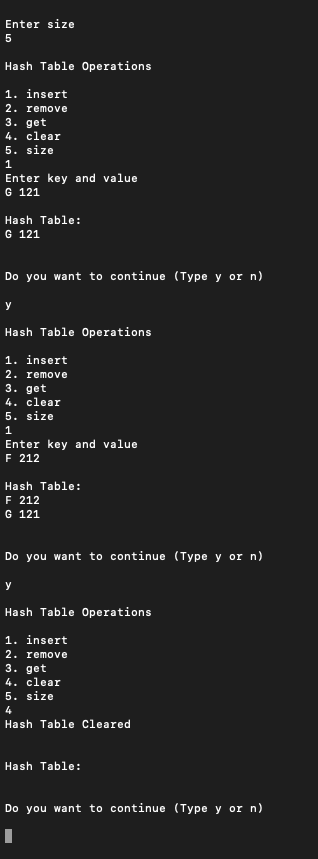Related Articles

# Java Program to Implement HashTables with Linear Probing

• Last Updated : 17 Jun, 2021

Hashing is a technique that is used to uniquely identify a specific object from a group of similar objects. Suppose an object is to be assigned a key to it to make searching easy. To store the key/value pair, one can use a simple array like a data structure where keys (integers) can be used directly as an index to store values. However, in cases where the keys are large and cannot be used directly as an index, one should use hashing. In hashing, large keys are converted into small keys by using hash functions. The values are then stored in a data structure called hash table. Linear Probing, It may happen that the hashing technique is used to create an already used index of the array. In such a case, we can search for the next empty location in the array by looking into the next cell until we find an empty cell. This technique is called linear probing.

There are three basic operations linked with linear probing which are as follows:

• Search
• Insert
• Delete

Implementation: Hash tables with linear probing by making a helper class and testing this in the main class.

Example

## Java

 `// Java Program to Implement Hash Tables with Linear Probing` `// Importing all classes from``// java.util package``// Importing all input output classes``import` `java.io.*;``import` `java.util.*;``// Importing Scanner class as in do-while``// inputs are entered at run-time when``// menu is popped to user to perform desired action``import` `java.util.Scanner;` `// Helper class - LinearProbingHashTable``class` `LinearProbingHashTable {``    ``// Member variables of this class``    ``private` `int` `currentSize, maxSize;``    ``private` `String[] keys;``    ``private` `String[] vals;` `    ``// Constructor of this class``    ``public` `LinearProbingHashTable(``int` `capacity)``    ``{``        ``currentSize = ``0``;``        ``maxSize = capacity;``        ``keys = ``new` `String[maxSize];``        ``vals = ``new` `String[maxSize];``    ``}` `    ``// Method 1``    ``// Function to clear hash table``    ``public` `void` `makeEmpty()``    ``{``        ``currentSize = ``0``;``        ``keys = ``new` `String[maxSize];``        ``vals = ``new` `String[maxSize];``    ``}` `    ``// Method 2``    ``// Function to get size of hash table``    ``public` `int` `getSize() { ``return` `currentSize; }` `    ``// Method 3``    ``// Function to check if hash table is full``    ``public` `boolean` `isFull()``    ``{``        ``return` `currentSize == maxSize;``    ``}` `    ``// Method 4``    ``// Function to check if hash table is empty``    ``public` `boolean` `isEmpty() { ``return` `getSize() == ``0``; }` `    ``// Method 5``    ``// Function to check if hash table contains a key``    ``public` `boolean` `contains(String key)``    ``{``        ``return` `get(key) != ``null``;``    ``}` `    ``// Method 6``    ``// Function to get hash code of a given key``    ``private` `int` `hash(String key)``    ``{``        ``return` `key.hashCode() % maxSize;``    ``}` `    ``// Method 7``    ``// Function to insert key-value pair``    ``public` `void` `insert(String key, String val)``    ``{``        ``int` `tmp = hash(key);``        ``int` `i = tmp;` `        ``// Do-while loop``        ``// Do part for performing actions``        ``do` `{``            ``if` `(keys[i] == ``null``) {``                ``keys[i] = key;``                ``vals[i] = val;``                ``currentSize++;``                ``return``;``            ``}` `            ``if` `(keys[i].equals(key)) {``                ``vals[i] = val;``                ``return``;``            ``}` `            ``i = (i + ``1``) % maxSize;` `        ``}` `        ``// Do-while loop``        ``// while part for condition check``        ``while` `(i != tmp);``    ``}` `    ``// Method 8``    ``// Function to get value for a given key``    ``public` `String get(String key)``    ``{``        ``int` `i = hash(key);``        ``while` `(keys[i] != ``null``) {``            ``if` `(keys[i].equals(key))``                ``return` `vals[i];``            ``i = (i + ``1``) % maxSize;``        ``}``        ``return` `null``;``    ``}` `    ``// Method 9``    ``// Function to remove key and its value``    ``public` `void` `remove(String key)``    ``{``        ``if` `(!contains(key))``            ``return``;` `        ``// Find position key and delete``        ``int` `i = hash(key);``        ``while` `(!key.equals(keys[i]))``            ``i = (i + ``1``) % maxSize;``        ``keys[i] = vals[i] = ``null``;` `        ``// rehash all keys``        ``for` `(i = (i + ``1``) % maxSize; keys[i] != ``null``;``             ``i = (i + ``1``) % maxSize) {``            ``String tmp1 = keys[i], tmp2 = vals[i];``            ``keys[i] = vals[i] = ``null``;``            ``currentSize--;``            ``insert(tmp1, tmp2);``        ``}``        ``currentSize--;``    ``}` `    ``// Method 10``    ``// Function to print HashTable``    ``public` `void` `printHashTable()``    ``{``        ``System.out.println(``"\nHash Table: "``);``        ``for` `(``int` `i = ``0``; i < maxSize; i++)``            ``if` `(keys[i] != ``null``)``                ``System.out.println(keys[i] + ``" "` `+ vals[i]);``        ``System.out.println();``    ``}``}` `// Main testing class``// Main Class for LinearProbingHashTableTest``public` `class` `GFG {``    ``// Main driver method``    ``public` `static` `void` `main(String[] args)``    ``{``        ``// Creating a scanner object``        ``// to take input from user``        ``Scanner scan = ``new` `Scanner(System.in);` `        ``// Display messages``        ``System.out.println(``"Hash Table Test\n\n"``);``        ``System.out.println(``"Enter size"``);` `        ``// maxSizeake object of LinearProbingHashTable``        ``LinearProbingHashTable lpht``            ``= ``new` `LinearProbingHashTable(scan.nextInt());` `        ``char` `ch;` `        ``// Do-while loop``        ``// Do part for performing actions``        ``do``        ``// Menu is displayed``        ``// LinearProbingHashTable operations performed as``        ``// per keys Users enter 'y' to continue 'n' if``        ``// entered by user , the program terminates` `        ``{``            ``// Menu``            ``// Display messages``            ``System.out.println(``"\nHash Table Operations\n"``);``            ``System.out.println(``"1. insert "``);``            ``System.out.println(``"2. remove"``);``            ``System.out.println(``"3. get"``);``            ``System.out.println(``"4. clear"``);``            ``System.out.println(``"5. size"``);` `            ``// Reading integer using nextInt()``            ``int` `choice = scan.nextInt();` `            ``// Switch case``            ``switch` `(choice) {` `            ``// Case 1``            ``case` `1``:` `                ``// Display message``                ``System.out.println(``"Enter key and value"``);``                ``lpht.insert(scan.next(), scan.next());``                ``// Break statement to terminate a case``                ``break``;` `            ``// Case 2``            ``case` `2``:` `                ``// Display message``                ``System.out.println(``"Enter key"``);``                ``lpht.remove(scan.next());``                ``// Break statement to terminate a case``                ``break``;` `            ``// Case 3``            ``case` `3``:` `                ``// Print statements``                ``System.out.println(``"Enter key"``);``                ``System.out.println(``"Value = "``                                   ``+ lpht.get(scan.next()));``                ``// Break statement to terminate a case``                ``break``;` `            ``// Case 4``            ``case` `4``:` `                ``lpht.makeEmpty();``                ``// Print statement``                ``System.out.println(``"Hash Table Cleared\n"``);``                ``// Break statement to terminate a case``                ``break``;` `            ``// Case 5``            ``case` `5``:` `                ``// Print statement``                ``System.out.println(``"Size = "``                                   ``+ lpht.getSize());``                ``break``;` `            ``// Default case``            ``// Executed when mentioned switch cases are not``            ``// matched``            ``default``:``                ``// Print statement``                ``System.out.println(``"Wrong Entry \n "``);``                ``// Break statement``                ``break``;``            ``}` `            ``// Display hash table``            ``lpht.printHashTable();` `            ``// Display message asking the user whether``            ``// he/she wants to continue``            ``System.out.println(``                ``"\nDo you want to continue (Type y or n) \n"``);` `            ``// Reading character using charAt() method to``            ``// fetch``            ``ch = scan.next().charAt(``0``);``        ``} ``while` `(ch == ``'Y'` `|| ch == ``'y'``);``    ``}``}`

Output:

Random action performed over Hash Table

• Size is entered as : 5
• Two key-value pairs are inserted
• G 121
• F 212
• Later Hash table is clearedAttention reader! Don’t stop learning now. Get hold of all the important Java Foundation and Collections concepts with the Fundamentals of Java and Java Collections Course at a student-friendly price and become industry ready. To complete your preparation from learning a language to DS Algo and many more,  please refer Complete Interview Preparation Course.

My Personal Notes arrow_drop_up